## 10.3. Programming the Python Script

1. If not yet existing, create the Python file. For this, select FileNew in the MATE menu bar and save the new file as `ApplicatorMacro.py` in the same folder as the other module files.

2. For the header of the file, take a look at other existing macro modules. What we need, besides the comment lines in #, is a line for importing the MeVis Python modules.

```# -----------------------------------------------------------------------------
## This file implements scripting functions for the ApplicatorMacro module
#
# \file    ApplicatorMacro.py
# \author  JDoe
# \date    01/2009
#
# -----------------------------------------------------------------------------

# MeVis module import
from mevis import *```
3. Then we need to add two functions, one for each scripting command

```def AdjustLength():
pass

def AdjustDiameter():
pass```
NoteIn Python, block structure is defined by indentation. Therefore it is important to indent the lines as shown in the code examples. In the MATE editor, this will happen automatically.
4. Let us have a look at the diameter adjustment. The diameter is given by the `diameter` field. This is written as follows:

```def AdjustDiameter():
diameter = ctx.field ("diameter").value```

To have both an effect on shaft and tip likewise, the diameter parameter of both must be set to the value of the `diameter` field. A look at the automatic panels of `SoCone` and `SoCylinder` shows that both modules offer a radius parameter.

Figure 10.13. Parameters for Diameter Setting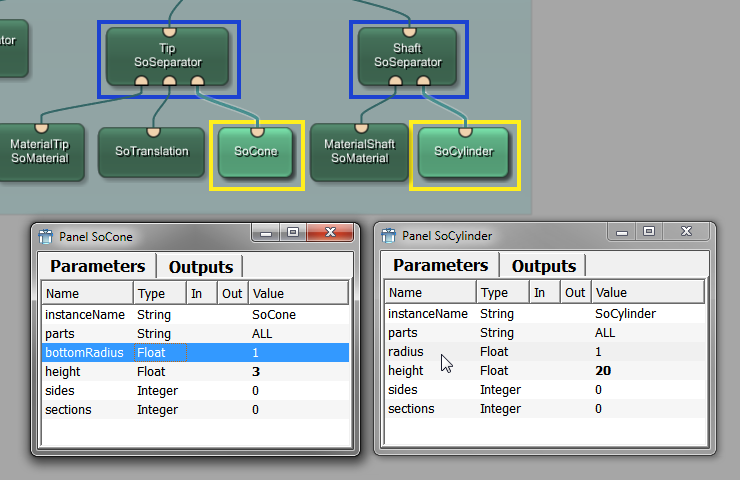These radius parameters need to be set to `diameter`:

```  ctx.field("SoCone.bottomRadius").value = diameter
ctx.field("SoCylinder.radius")  .value = diameter```

As the radius is half the diameter, a correcting factor of 0.5 has to be added to the diameter equation.

```def AdjustDiameter():
diameter = ctx.field("diameter").value * 0.5

ctx.field("SoCone.bottomRadius").value = diameter
ctx.field("SoCylinder.radius")  .value = diameter```
5. To test if the diameter adjusting works, add a `SceneInspector` module to the network and connect its input to the output of your `ApplicatorMacro` module. Double-click the `SceneInspector` to open its viewer. When you change the diameter setting of the macro, the diameter of the applicator is changed accordingly.

Figure 10.14. Changing the Diameter of the Applicator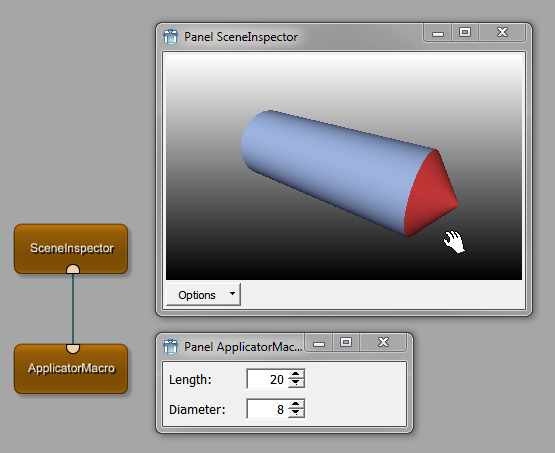6. Adjusting the length is a bit more complicated. The length change should have the following effects:

• The `length` parameter gives the overall length.

• Only the shaft should be extended, not the tip.

• The adjustment should be done in a way that the point of the tip is not translated, that is that the tip points to the same position as before. Therefore, we need to increase the applicator length in the direction away from the tip.

We can define an overall length, a tip length and a shaft length. They can be calculated as follows:

```def AdjustLength():
overallLength = ctx.field("length").value
tipLength     = ctx.field("SoCone.height").value

shaftLength   = overallLength - tipLength```

The original translation factor for the tip (which is the relevant factor) was given by half the shaft length (“10”) plus half the tip length (“1.5”). This can be written in a general way.

`  tipTranslation = shaftLength*0.5 + tipLength*0.5`

The shaftLength defines the height of the `SoCylinder` cone to

`  ctx.field("SoCylinder.height").value = shaftLength`

The resulting code lines for the length adjustment look as follows:

```def AdjustLength():
overallLength  = ctx.field("length").value
tipLength      = ctx.field("SoCone.height").value

shaftLength    = overallLength - tipLength
tipTranslation = shaftLength*0.5 + tipLength*0.5

ctx.field ("SoCylinder.height").value = shaftLength```

Add this code to the Python script, save, and reload the definition. A test shows a funny effect: the shaft length is changed independently of the tip.

Figure 10.15. Strange Behavior of the ApplicatorMacro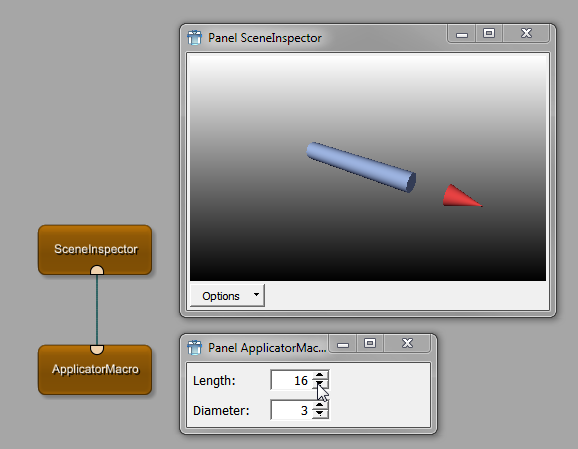This is due to not having connected the calculated `tipTranslation` with the `TranslationTip` module yet.

7. To solve this problem, add the `SoComposeVec3f` module to the internal network of the macro and assign to its translation in y direction the calculated value tipTranslation. Since SoComposeVec3f supports an arbitrary number of elements on x,y,z, we have to use `setListValue`.

`  ctx.field("SoComposeVec3f.y").setListValue([tipTranslation])`
8. In a last step, this translation needs to be connected to the tip's `SoTranslation` module via a parameter connection in the network.

Figure 10.16. Adding the Correct Tip Translation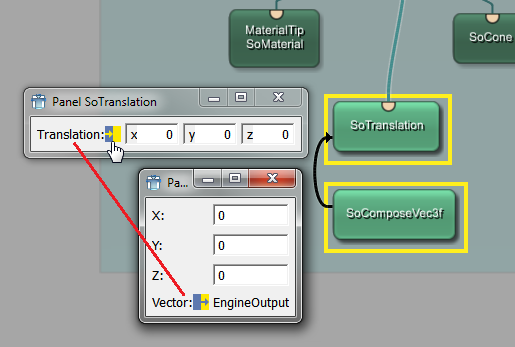Here the network and complete Python script of the ApplicatorMacro example:

Figure 10.17. Complete ApplicatorMacro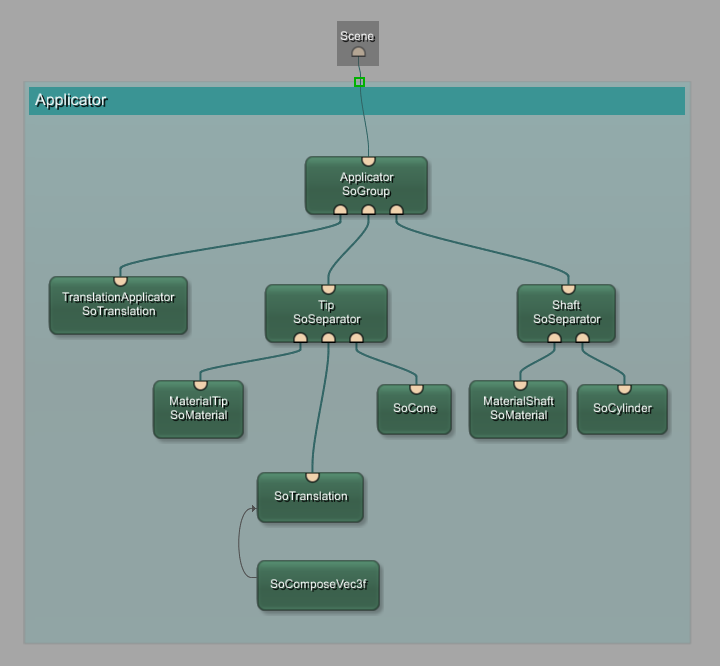```# -----------------------------------------------------------------------------
## This file implements scripting functions for the LocalFileName module
#
# \file    ApplicatorMacro.py
# \author  JDoe
# \date    01/2009
#
# -----------------------------------------------------------------------------

# MeVis module import
from mevis import *

def AdjustDiameter():
diameter = ctx.field("diameter").value * 0.5

ctx.field("SoCone.bottomRadius").value = diameter
ctx.field("SoCylinder.radius")  .value = diameter

def AdjustLength():
overallLength  = ctx.field("length").value
tipLength      = ctx.field("SoCone.height").value

shaftLength    = overallLength - tipLength
tipTranslation = shaftLength*0.5 + tipLength*0.5

ctx.field("SoCylinder.height").value = shaftLength
ctx.field("SoComposeVec3f.y") .setListValue([tipTranslation])```